Register for Maths, Science, English, Reasoning Olympiad Exams Register here | Book Free Demo for Live Olympiad Classes here | Check Olympiad Exam Dates here | Buy Practice Papers for IMO, IOM, HEO, IOEL etc here | Login here to participate in all India free mock test on every Saturday

# Measurement-Money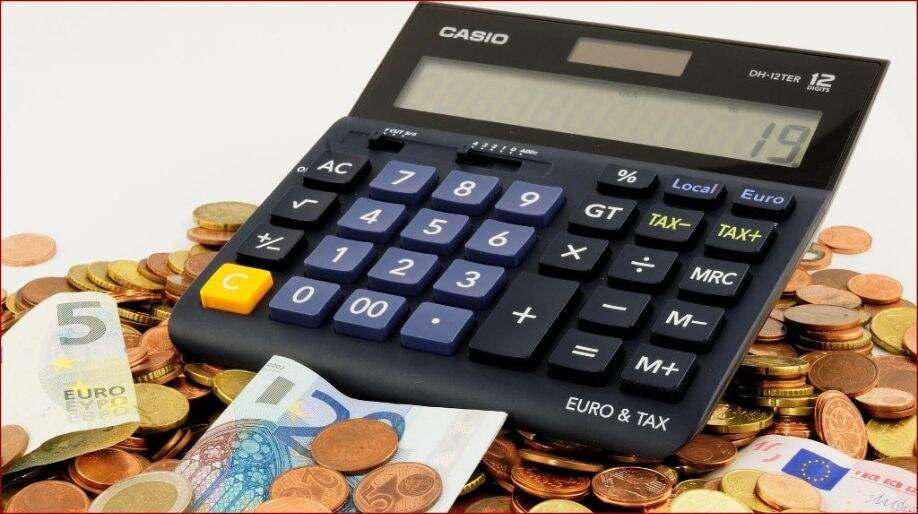Measurement-Money

Money is useful for purchasing things like chocolates, pencils, vegetables, etc. In India we have currency in rupees and paisa.

Here are some coins and notes we use in India: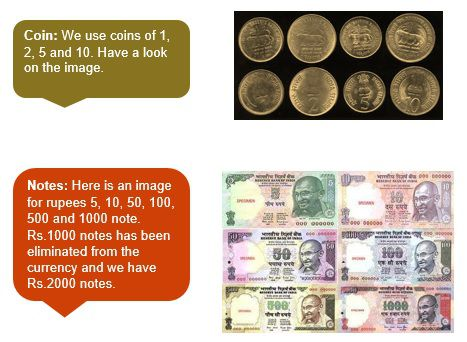Conversion of Money: Here we will learn the ways to convert rupees into paisa and vice versa.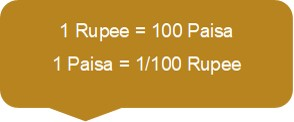Example 1: Convert 5.09 rupees into paisa

Solution: We know that 1 rupee = 100 paisa

Therefore, 5.09 rupee = 5.09×100 = 509 paisa

Example 2: Convert 78 paisa into rupees

Solution: We know that 1 paisa = 1/100 rupees

Therefore, 78 paisa = 78/100 = 0.78 rupee

Try These:

• Convert the following amounts into paisa:
1. 8.10
2. 0.95
• 12.24

• Convert the following amounts into rupees:
1. 775 paisa
2. 38 paisa
• 3099 paisa

Some Important Terms and Formulae

• Cost Price: The price at which an article is purchased, is called its cost price. It is denoted by CP.

• Selling Price: The price at which any article is sold, is called its selling price. It is denoted by SP

• Profit: When the SP is more than CP, profit exists. It is defined as the difference between SP and CP.

• Loss: When the CP is more than SP, then the article is sold at a loss. It is the difference between CP and SP.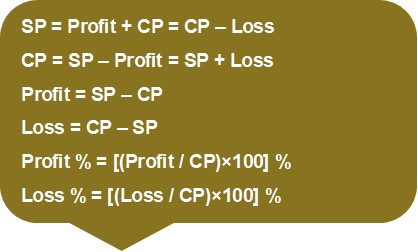Example: Radhika bought a TV for Rs 23,500 and sold at a loss of Rs 4,780. Find the selling price.

Solution:  CP = Rs 23,500

Loss = Rs 4,780

SP = CP – Loss = Rs (23,500 – 4,780) = Rs 18,720

Practice Exercise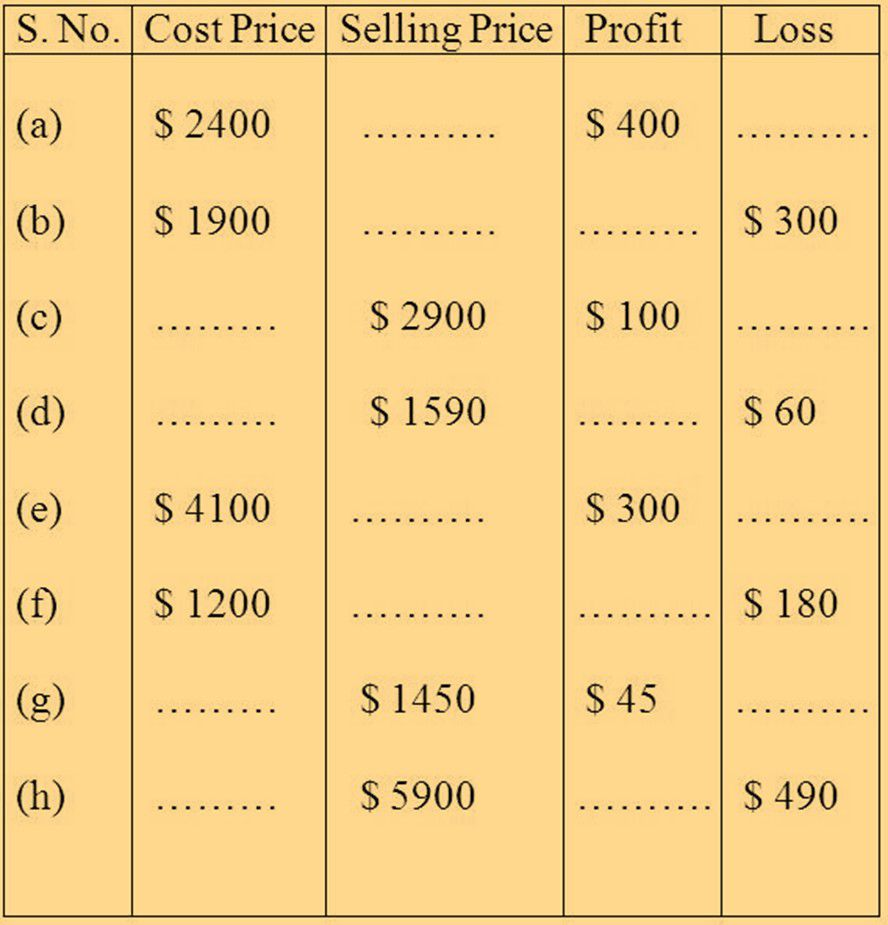Recap

• 1 rupee = 100 paisa
• Profit = Selling price – Cost Price
• Loss = Cost Price – Selling Price
• Profit % = [(Profit / CP)×100] %
• Loss % = [(Loss / CP)×100] %

## Quiz for Measurement-Money

 Q.1 Express the money in the short form 17 rupees 75 paise: a) Rs. 17.57 b) Rs. 77.15 c) Rs. 17.75 d) Rs. 15.77
 Q.2 Express the money in the short form 195 rupees 15 paise a) Rs. 19.51 b) Rs. 195.15 c) Rs. 155.95 d) Rs. 195.25
 Q.3 Express the money in the long form Re 0.95 a) 95 rupees b) 1 rupees 95 paise c) 95 paise d) 950 paise
 Q.4 Express the money in words Rs. 49.36 a) Forty six rupees and ninety three paise b) Thirty six rupees and forty nine paise c) Forty nine rupees and thirty six paise d) Sixty three rupees and forty nine paise
 Q.5 Convert the money into paise:- Rs. 78.12 a) 7821 paise b) 1278 paise c) 7812 paise d) 7128 paise
 Q.6 Convert the money into paise 97 rupees 54 paise a) 9754 paise b) 9547 paise c) 9647 paise d) 9457 paise
 Q.7 Shaila bought a bag of Rs. 50 and a battery for Rs. 6.50. Based on this information, answer the next two questions. How much did she pay? a) Rs. 56.00 b) Rs. 65.20 c) Rs. 55.20 d) Rs. 56.50
 Q.8 If a notebook costs Rs. 5, a pencil Rs. 1.50, a toffee Rs. 0.50 and one pack of biscuit Rs. 4.50. Based on this information, answer the next five questions. Rina had Rs 44.50, Raman had Rs 85.50 and Venu had Rs 36.00. How much money did they have in all? a) Rs. 168 b) Rs. 166 c) Rs. 166.50 d) Rs. 165
 Q.9 If a notebook costs Rs. 5, a pencil Rs. 1.50, a toffee Rs. 0.50 and one pack of biscuit Rs. 4.50. Based on this information, answer the next five questions. What is the cost of two notebooks, 1 pencil and 1 toffee? a) Rs. 10.50 b) Rs. 12 c) Rs. 11.50 d) Rs. 11
 Q.10 If an ice-cream costs Rs. 15.50 and you have Rs. 35, then how many ice-creams you can buy? a) 4 b) 1 c) 3 d) 2